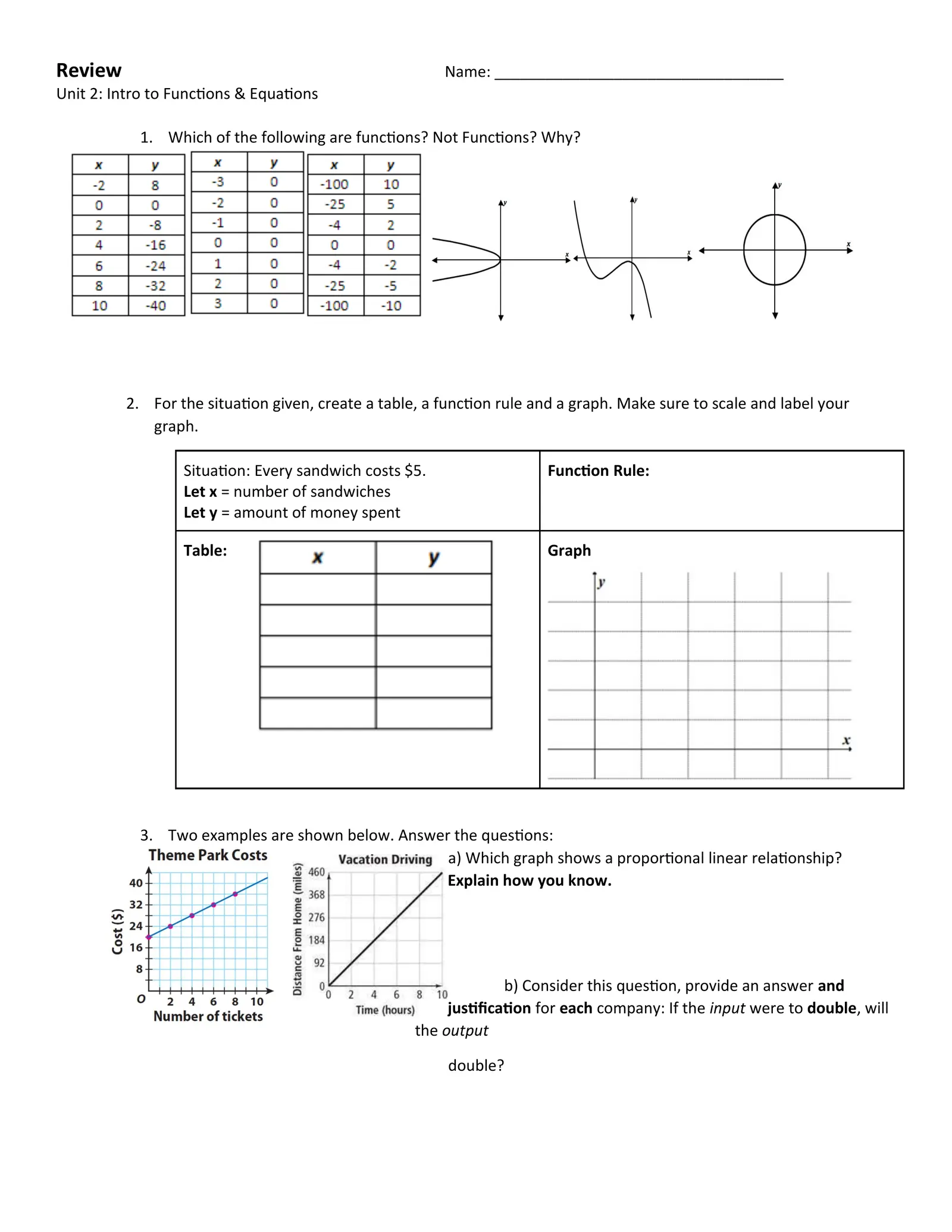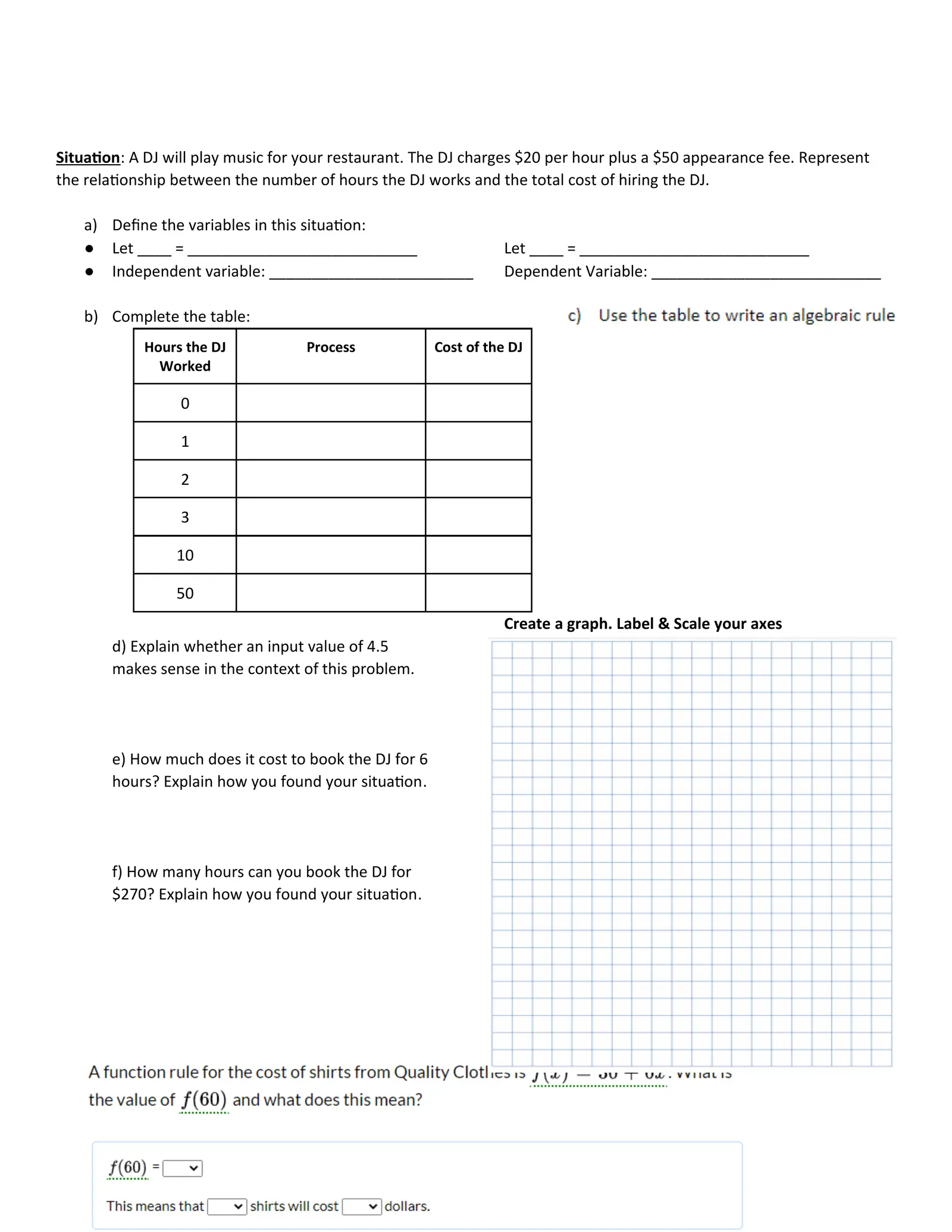# Unit 2 End of Unit Practice

Created
Best for live in-class or video conferencing lessonsStart teacher-led lessonReview Name: __________________________________ Unit 2: Intro to Functions & Equations 1. Which of the following are functions? Not Functions? Why? 2. For the situation given, create a table, a function rule and a graph. Make sure to scale and label your graph. Situation: Every sandwich costs \$5. Function Rule: Let x = number of sandwiches Let y = amount of money spent Table: Graph 3. Two examples are shown below. Answer the questions: a) Which graph shows a proportional linear relationship? Explain how you know. b) Consider this question, provide an answer and justification for each company: If the input were to double, will the output double?

1
You need to do the graph on your own paper4) Answer these questions about Metacognition: a) What is metacognition? b) How is metacognition helpful in learning? c) Give some examples of metacognition questions that you can ask yourself while working on problems. 5) Consider the function rule f ( x )=2 x−3 a. Find f (4) b. Find f (−2) b. Show or explain how the equation 2 x−3=53 can be solved using the “undoing process”. Report a solution to the equation 6) Consider the function rule f ( x )=−3 x +10 a) Find f (4) b) Find f (−2) c. Show or explain how the equation −3 x +10=46 can be solved using the “undoing process”. Report a solution to the equation 3+ x 7) Consider the rule h (x )= 2 a) Find f (3) b) Find f (12)Situation: A DJ will play music for your restaurant. The DJ charges \$20 per hour plus a \$50 appearance fee. Represent the relationship between the number of hours the DJ works and the total cost of hiring the DJ. a) Define the variables in this situation: ● Let ____ = ___________________________ Let ____ = ___________________________ ● Independent variable: ________________________ Dependent Variable: ___________________________ b) Complete the table: Hours the DJ Process Cost of the DJ Worked 0 1 2 3 10 50 Create a graph. Label & Scale your axes d) Explain whether an input value of 4.5 makes sense in the context of this problem. e) How much does it cost to book the DJ for 6 hours? Explain how you found your situation. f) How many hours can you book the DJ for \$270? Explain how you found your situation.

Don't worry about completing this one